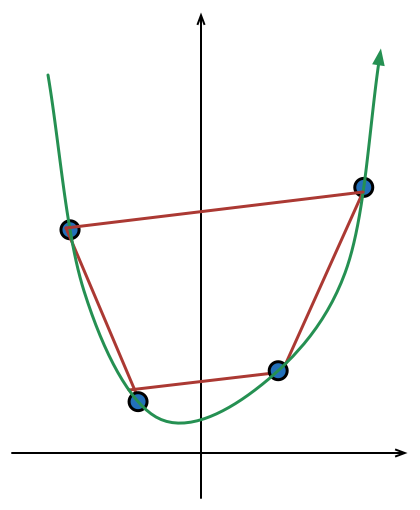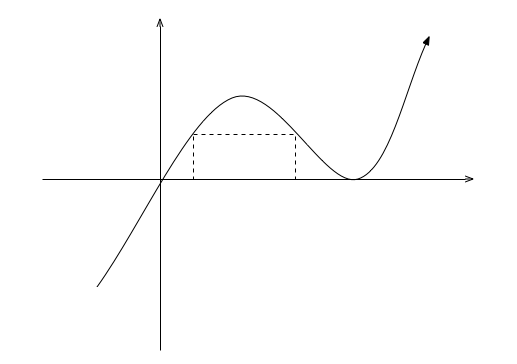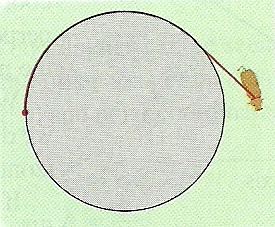Calculus

# Calculus Warmups: Level 5 ChallengesConsider a curve $y=ax^2 +bx+c$ with $a, b, c \in \mathbb{N}$ which passes through four points $A(-2,3),B(-1,1),C(\alpha ,\beta ),D(2,7)$.

All these points are taken in given order for constructing a convex quadrilateral, which has maximum possible area.

Find minimum possible value of $a+b+c+2\alpha +4\beta.$

A rectangle's bottom is at $y=0$while its top corners are on the curve $y=x{ (x-1) }^{ 2 }$ between $x=0$ and $x=1$. The maximum area of this rectangle can be expressed as

$\dfrac { a\sqrt { a } -b }{ c\sqrt { d } }$

where $a$ and $d$ are prime numbers. What is the sum $a+b+c+d?$

(Don't count $a$ twice)

Let

$f(x) = \sin^{2} x - \frac{1}{2} \sin2x \times \sin^{2}x + \frac{1}{3} \sin3x \times \sin^{3}x - \ldots$

Then $\displaystyle f \left( \frac{\pi}{12} \right)$ can be written as

$\tan^{-1} \left( \dfrac{ a - \sqrt{b}}{c} \right)$

where $b$ is square free & $a,b,c \in\mathbb N$. Find the value of $a+b+c$.A cow is tied to a silo with radius $r$ by a rope just long enough to reach the opposite end of the silo.

Find the area available for grazing by the cow.

Enter the answer for $r=10$, rounded to the nearest hundredth.

$\displaystyle \lim _{ n\rightarrow \infty }{ \frac { \displaystyle \sum _{ r=1 }^{ { 2 }^{ n-1 }-1 }{ \tan ^{ 2 }{ \left (\frac { r\pi }{ { 2 }^{ n } } \right ) } } }{ { 4 }^{ n } } }$

For positive integer $n$, the limit evaluates to $\dfrac a b$ for coprime positive integers $a,b$. What is the value of $a+b$?

×